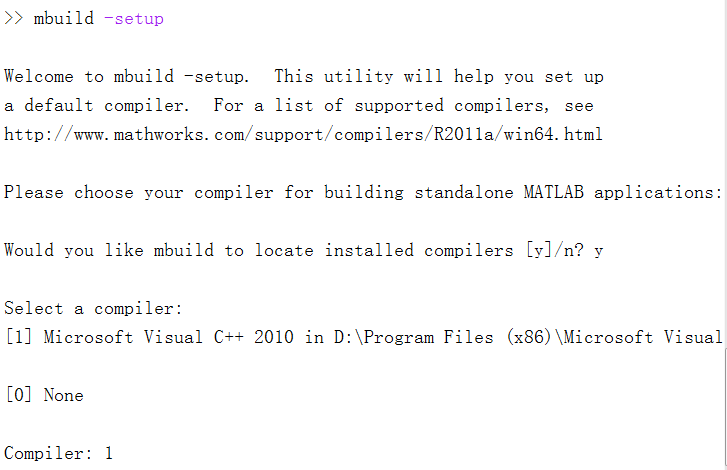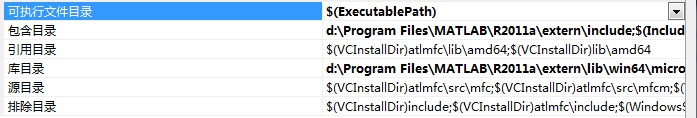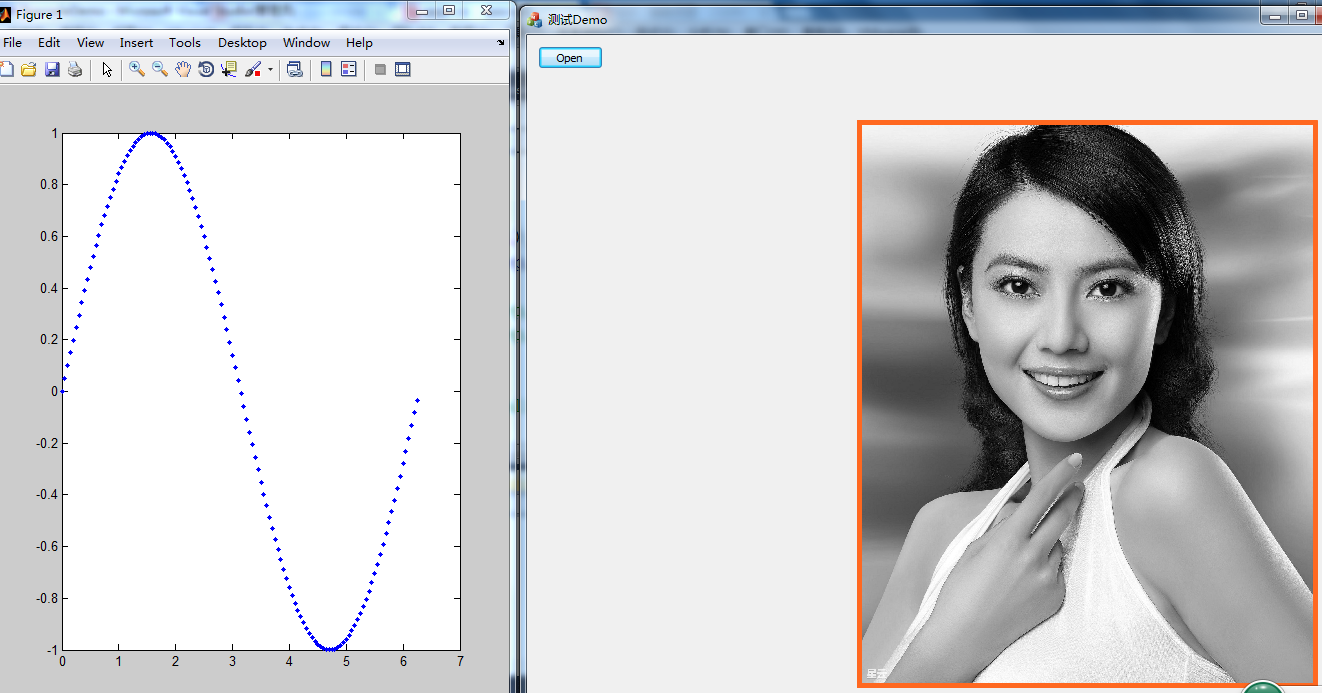• ## c++调用matlab程序

万次阅读 2015-05-22 17:11:03
Matlab 与c++混合编程方法 ...不过我认为学习c++与matlab混合编程一般是通过c++调用matlab函数，因为matlab具有强大的数学函数库，然而vc++具有界面设计灵活的优点，因此这里只介绍通过vc++调用ma

Matlab 与c++混合编程方法

开发环境：win7 64位+vs2010+matlab

如果想要让matlab调用c++的函数类，则需要把c++编译成mex文件，使用这个方法可以实现混合调试。不过我认为学习c++与matlab混合编程一般是通过c++调用matlab函数，因为matlab具有强大的数学函数库，然而vc++具有界面设计灵活的优点，因此这里只介绍通过vc++调用matlab的方法。

(1)环境配置搭建

matlab配置：开始前matlab需要先安装两个编译插件，这个安装直接在matlab命令窗口中敲入分别输入命令：

>>mex -setup

>>mbuild –setup安装过程中要么直接回车，要么选择相关的编译器。

vc++项目配置：安装完毕之后，配置c++程序中的包含目录、库目录这个对应于你的电脑matlab的安装位置分别把.h和lib文件指向..\extern\include、..\extern\lib\win64\microsoft.除此之外还要在”链接器-》附加依赖项 “ 中加入调用eigin所需的库libmx.lib、 libmat.lib、libeng.lib，以及调用mwArray所需的类的库mclmcrrt.lib，mwArray是连接c++与matlab所需的 矩阵类，如果不懂这个类的使用需要先网上查看一下用法。同时还要在系统坏境变量中，添加matlab的动态链接库.dll文件所在的位置，这个就在matlab安装位置的bin目录下。

(2)程序调用

接着就要开始学习如何通过c++调用matab的函数库了，这个调用方法有两个：

第一种方法：在c++中直接调用matlab的计算引擎eigin。在调用的文件中添加eigin头文件：

#include <engine.h>

然后在程序想要引用matlab的地方调用eigin函数：

Engine *ep;ep=engOpen(NULL);engEvalString(ep,"x=0:0.05:2*pi;y=sin(x);plot(x,y,'.b')");

我这里是通过c++调用matlab，来绘制一个正玄函数作为例子，在engEvalString函数就是输入命令函数的代码，当然这个函数可以每次在输入的字符串当中只有一条命令，然后多次调用engEvalString函数，你也可以直接把所有的matlab函数代码直接当做一个字符串，输入engEvalString函数中。测试一下调用结果：我在例子中通过mfc对话框的open按钮，打开读取图片，并且调用matlab函数的绘制函数，可以看到同时出现了mfc与matlab绘制窗口的共存。我最喜欢用matlab与c++混合编程的原因就是这个，c++逻辑调试比较难，但是如果由matlab显示c++程序运行中一些数据的变化结果进行调试，那就相当爽了。

这种调用eigin的方法缺点在于要求运行程序的电脑中装有matlab，这个方法我比较不喜欢，因为在公司上班的时候，经常要把自己的demo结果发给其他人测试，而其它人的电脑上没有装matlab，这样就调用不了matlab的eigin

第二种方法：使用把matlab函数生成为动态链接库，然后在c++中调用该动态链接库：这里测试一下通过c++输入数据给matlab，这些数据是一个图片的其中一个通道数据，然后在matlab中显示该单通道图片，最后把数据返回给c++。采用这种方法就要用到mwArray类实现数据传输了。

matlab图片数据显示函数

[c++] view
plaincopy

function [ output_args ] = test( input_args )
[n m]=size(input_args);
A=uint8(input_args');
imshow(A);
output_args=[3,4,5];
end

然后在matlab的命令窗口输入如下命令：

>>mcc -W cpplib:plotsintest -T link:lib test.m

将生成名字为：plotsintest的动态链接库、静态链接库、头文件，最后再vs中，属性》链接器》附加依赖项》

plotsintest.lib
mclmcrrt.lib
libmx.lib
libmat.lib
libeng.lib

然后在c++中加入头文件plotsintest.h，然后在程序运行的地方调用代码为：

[c++] view
plaincopy

if( ! mclInitializeApplication(NULL,0) )
{
fprintf(stderr, "Could not initialize the application.\n");
exit(1);
}

if (! plotsintestInitialize() )
{
fprintf(stderr,"Could not initialize the library.\n");
exit(1);
}
int width=m_pOrignImage->GetWidth();
int heigth=m_pOrignImage->GetHeight();
double *r=new double[heigth*width];
for (int i=0;i<heigth*width;i++)
{
r[i]=((BYTE*)lockData.Scan0)[i*4];
}
mwArray X(width,heigth,mxDOUBLE_CLASS);//定义输入X;
mwArray Y;//(width,heigth,mxDOUBLE_CLASS);//定义输入Y;
X.SetData(r,heigth*width);
test(1,Y,X);
plotsintestTerminate();
mclTerminateApplication();

最后显示结果为：可以看到，mfc对话框读取显示图片，同时matlab出现了绘制figure窗口。原文地址：http://blog.csdn.net/hjimce/article/details/44904271    作者：hjimce

展开全文• 学习c++与matlab混合编程一般是通过c++调用matlab函数，因为matlab具有强大的数学函数库，然而vc++具有界面设计灵活的优点，下面这篇文章主要给大家介绍了关于在ubuntu系统下C++调用matlab程序的方法，需要的朋友...
• 之前已经跟大家介绍了在ubuntu系统下C++调用matlab程序的方法，需要的朋友们可以参考这篇文章，本文将给大家介绍关于windows下C++调用matlab程序的相关内容，分享出来供大家参考学习，下面话不多说了，来一起看看...
• 此处采取的方法是 使用matlab函数生成为动态链接库，然后在c++调用该动态链接库。 主要参考博客：http://blog.csdn.net/u010910436/article/details/45919457与http://www.codesec.net/view/432735.html在期间...
此处采取的方法是 使用matlab函数生成为动态链接库，然后在c++中调用该动态链接库。
主要参考博客：http://blog.csdn.net/u010910436/article/details/45919457与http://www.codesec.net/view/432735.html

可以使用 >>!mcc命令来查看mcc的用法

在期间遇到的问题：

2.在编译完成之后，将头文件，静态库文件，动态库文件都不配置完成之后，还需要配置MATLAB的扩展头文件和库文件路径，此时需要注意MATLAB和C++需要同时为W32或者是x64，然后加入
libeng.lib
libmat.lib
libmex.lib
libmx.lib
mclmcrrt.lib
mclmcr.lib
这些库文件便可以成功编译。
展开全文c语言
• 本文件是C++调用MATLAB程序，平台是VS2012和MATLAB 2017，这里的平台不能随意更换，因为存在兼容性问题。程序整体已经通过测试。相关的混合编程理论可见我的博客文章。
• 前言之前已经跟大家介绍了在ubuntu系统下C++调用matlab程序的方法，需要的朋友们可以参考这篇文章，本文将给大家介绍关于windows下C++调用matlab程序的相关内容，分享出来供大家参考学习，下面话不多说了，来一起看...
前言之前已经跟大家介绍了在ubuntu系统下C++调用matlab程序的方法，需要的朋友们可以参考这篇文章，本文将给大家介绍关于windows下C++调用matlab程序的相关内容，分享出来供大家参考学习，下面话不多说了，来一起看看详细的介绍吧。实验平台：matlab R2016b   VS2013思路：1. 设置matlab的编译器，使用外部的VC或者gcc等编译器。2. 编译m文件成dll3. 设置VS的Include路径和lib链接库的路径4. 编写C++调用dll步骤：1.    设置matlab的编译器在命令行窗口下，输入并执行如下命令：mex –setup在出现的编译器中，选择VS2013然后在输入命令：mbuild –setup 同样选择VS20132.    编写.m文件如下函数时完成图像的分割功能，第一个参数是图像的文件名(路径)，第二个参数是分割图像阈值的大小；完成分割后，将图像保存为result.bmp;返回值则是原图像的数据；function imagedata=improcess(filename,threshold);imagedata=double(imread(filename));newbuf=imagedata;[M N]=size(imagedata);for i=1:1:Mfor j=1:1:Nif imagedata(i,j)>thresholdnewbuf(i,j)=255;elsenewbuf(i,j)=0;endendendimwrite(uint8(newbuf),'result.bmp');return;3.    编译.m文件mcc -W cpplib:pr_lib -T link:lib improcess.m解释：其中-W是控制编译之后的封装格式；cpplib，是指编译成C++的lib；cpplib冒号后面是指编译的库的名字；-T表示目标，link:lib表示要连接到一个库文件的目标，目标的名字即是.m函数的名字。编译完成之后，pr_lib.h pr_lib.libpr_lib.dll这三个文件时我们在c++中调用所需要的；这三个文件和我们用c++编写dll时，生成的三个文件时对应的；VS中的调用步骤1.    设置VC环境将matlab的头文件路径和对应的库文件路径包含到VS；在VS中，点项目属性然后在‘VC++目录目录'选项卡中，将包含目录的最后一行，添加matlab相关的头文件；如我的matlab对应的路径为：........\MATLAB\R2016b\extern\include........\MATLAB\R2016b\extern\include\win64将库目录，将matlab相关的静态链接库的路径加入其中：........\MATLAB\R2016b\extern\lib\win32\microsoft将链接器->输入：libeng.liblibmat.liblibmex.libmclmcrrt.libpr_lib.lib2.    新建一个基于控制台的hello World程序；2.1    添加必须的头文件和必须的静态链接库如果在上面的链接库中添加了lib， 下面只需要加入头文件就可以了。#pragma comment(lib,"mclmcrrt.lib")#pragma comment(lib,"libmx.lib")#pragma comment(lib,"libmat.lib")#include "matrix.h"#include "stdafx.h"#include #include "pr_lib.h"2.2    将matlab编译生成的pr_lib.h pr_lib.libpr_lib.dll文件拷贝到工程目录下；并将头问价和静态链接库添加到工程中：#pragma comment(lib,"pr_lib.lib")#include "pr_lib.h"2.3    编辑main函数，调用improcess函数；int main(int argc, char* argv[]){//初始化， 在C++调用matlab时，必须要进行初始化。if( !pr_libInitialize()){printf("Could not initialize !");return -1;}char f_name="lenna.pgm";//必须将lenna.pgm图像，拷贝到工程目录下mwArray file_name(f_name);//'lenna.pgm'mwArray m_threshold(1,1, mxDOUBLE_CLASS);m_threshold(1,1)=128;//阈值为128mwArray ImageData(512,512, mxDOUBLE_CLASS);improcess(1,ImageData,file_name,m_threshold);//1,表示返回值的个数，ImageData用于接收返回值printf("\n图像处理结束,已经图像以阈值128分割开！\n");double *resultdata=new double[512*512];ImageData.GetData(resultdata,512*512);printf("\n已获得图像数据...\n");for(int i=0;i<512;i++){for(int j=0;j<512;j++){printf("%0.1f ",resultdata[512*i+j]);}printf("\n");}delete []resultdata;// 后面是一些终止调用的程序// terminate the libpr_libTerminate();return 0;}解析：从上面的程序我们可以看出，c++与matlab函数数据传递是借助matlab定义的mwArray类完成的！该类支持的数据类型有：/*typedef enum{mxUNKNOWN_CLASS = 0,mxCELL_CLASS,mxSTRUCT_CLASS,mxLOGICAL_CLASS,mxCHAR_CLASS,mxVOID_CLASS,mxDOUBLE_CLASS,mxSINGLE_CLASS,mxINT8_CLASS,mxUINT8_CLASS,mxINT16_CLASS,mxUINT16_CLASS,mxINT32_CLASS,mxUINT32_CLASS,mxINT64_CLASS,mxUINT64_CLASS,mxFUNCTION_CLASS,mxOPAQUE_CLASS,mxOBJECT_CLASS}*/同时我们应注意：mwArray类定义的对象数组，其坐标仍然是从1开始，而不是像c++那样，从0开始！mwArray的更详细的用法，可以参看mclcomclass.h总结以上就是这篇文章的全部内容了，希望本文的内容对大家的学习或者工作能带来一定的帮助，如果有疑问大家可以留言交流，谢谢大家对脚本之家的支持。
展开全文• 在vs2010中新建一个C++控制台工程，添加依赖项：（请根据自己的matlab安装目录更改） 1)、在include files中添加： E:\matlab\2012a-launch\extern\include 2)、在library fi...
以64 bit win7下的matlab 2012a(64bit)和vs2010为例：

在vs2010中新建一个C++控制台工程，添加依赖项：（请根据自己的matlab安装目录更改）1)、在include files中添加：

E:\matlab\2012a-launch\extern\include2)、在library files中添加：

E:\matlab\2012a-launch\extern\lib\win64\microsoft3)、在input中添加：

libmx.lib;libmex.lib;libeng.lib;（注意：将vs2010的运行环境从 win32 改成 x64）

示例程序：

#include <iostream>
#include <cmath>
#include <string>

#include <engine.h>

using namespace std;

int main(int argc, char** argv) {
Engine* m_pEngine;
m_pEngine = engOpen(NULL);
if( m_pEngine == NULL ) {
cout<<"error!"<<endl;
exit(-1);
}
engEvalString(m_pEngine, "x=0:0.01:2*pi;");
engEvalString(m_pEngine, "y=sin(x);");
engEvalString(m_pEngine, "figure; plot(x,y,'g');");

system("pause");
engClose(m_pEngine);

return 0;
}

报错：
1）、无法解析的外部符号 _engClose，该符号在函数 _main 中被引用 。
解决：换到x642)、无法启动此程序，因为计算机中丢失libeng.dll。尝试重新安装该程序以解决此问题。
解决：E:\Program Files\MATLAB\R2013a\bin\win64 加入系统环境变量

参考：
 fourfire.c++，matlab混合编程[EB/OL].[2014-03-11].http://blog.sina.com.cn/s/blog_8e6bfecf0100y5ba.html
  justinlm.Visual Studio 2010 为C/C++添加外部库的方法[EB/OL].[2014-03-11]http://www.docin.com/p-156218299.html
 hwby199106wHow_to_use_Matlab_Engine_in_Visual_Studio[EB/OL].[2014-03-11].http://www.doc88.com/p-458271665677.html

转载于:https://www.cnblogs.com/ccdc/p/3593275.html
展开全文• 前言之前已经跟大家介绍了在ubuntu系统下C++调用matlab程序的方法，需要的朋友们可以参考这篇文章，本文将给大家介绍关于windows下C++调用matlab程序的相关内容，分享出来供大家参考学习，下面话不多说了，来一起看...
• 本篇文章主要介绍混合编程——C++调用MATLAB程序，以及常用问题解决，并给出通过测试的示例程序。 目录： C++调用MATLAB程序方法 示例程序 常用问题解决 程序下载 C++调用MATLAB程序方法 matlab mex编译器...C 混合编程
• ## C/C++调用matlab程序

千次阅读 2013-08-14 21:02:42
C/C++调用MATLAB程序可以通过多种方式： 生成独立运行的MATLAB程序是使用MATLAB编译器编译m文件最常用的一种方式。通过将m文件编译为可独立运行的可执行文件，为用户提供了一种最简单的发布MATLAB程序的方法。 Ø...
• C++调用MATLAB程序，以及常用问题解决。欢迎使用Markdown编辑器新的改变功能快捷键合理的创建标题，有助于目录的生成如何改变文本的样式插入链接与图片如何插入一段漂亮的代码片生成一个适合你的列表创建一个表格...混合编程
• 前言最近因为工作的需要在研究C++怎么调用matlab程序，发现网上的资料较少，所以将自己学习的内容总结分享出来，下面话不多说了，来一起看看详细的介绍吧。实验平台：ubuntu matlab R2016b g++步骤：1. 设置matlab的...
• ## C/C++调用Matlab程序

千次阅读 2016-04-19 09:49:44
在用C写程序时，有时会遇到数值计算问题，如矩阵的计算、优化问题、解微分方程等等。这些数值计算问题如果自己来实现的话，一来太费时间，二来自己的实现方案...在C程序调用matlab函数即可轻松解决问题。 在进行联
• 1、头文件添加 #include &...5、以下程序启动matlab  Engine* ep = engOpen(NULL); 6、之后便可以使用matlab了，使用格式如下： engEvalString(ep, "。。。。。。。。。。。"); 双引号里面...
• C# C++调用MATLAB C# C++调用MATLAB C# C++调用MATLAB C# C++调用MATLAB
• 在项目中，遇到需要用C++调用Matlab程序来做图像处理，这里记录下整个过程。以供遇到类似问题的读者作参考。此问题棘手的原因主要有在Matlab编写的项目程序中用到了很多图像处理封装库，经过多次尝试，最终在Visual ...qt linux 算法
• 在vs2010中新建一个C++控制台工程，添加依赖项：（请根据自己的matlab安装目录更改）     1)、在include files中添加： E:\matlab\2012a-launch\extern\include   2)、在library fi
• vs2013平台下实现c++调用matlab代码，具有通用性
• c++调用matlab函数，c++程序有很多种方法调用Matlab中的函数，但是我最喜欢的方法是直接调用Matlab的动态库，这样生成的程序在没有安装Matlab的机子上也可以运行。
• [MATLAB]C++调用MATLAB引擎 MATLAB版本：R2018b 操作系统：Window 10 1 需求分析 在C++开发过程中，可能需要C++和MATLAB混合编程，比如：别人只会MATLAB，需要和我们的C++一起运行；或者有部分MATLAB/Simulink开发...cmake
• 主要为大家详细介绍了C++调用Matlab函数求特征值，具有一定的参考价值，感兴趣的小伙伴们可以参考一下
• 平台：XP,Code::Blocks 10.05(包含gcc 4.4.1),matlab 2010b方式：C++调用matlab引擎设置步骤：(一)系统变量：path中添加MinGW\bin; MATLAB\runtime\win32;MATLAB\bin; MATLAB\bin\win32, 即MinGW的bin目录，matlab的...
• visual C++ 调用matlab 引擎设置
• C++调用Matlab的VS2010项目源代码 提前书案MATLAB并进行了mbuild -setup的环境设置，在VS项目里设置自己的MATLAB的库目录和包含路径。 里面包含三个项目：二个基于C++调用MATLAB的DLL动态链接库，一个基于C++调用......

# c++调用matlab程序c++ 订阅matlab 订阅